# Lions Weekly: January 12, 2022# A Note From Mr. Sandefur

Lion Family,

Among the many things that I am thankful for this week is the ability to be with our students as the weather has cooperated allowing us to kick off our second semester. We are truly grateful for the support of our families last week as we made decisions in order to keep members of our school community as safe as possible. I am personally grateful that we are back in the swing of activities throughout our campus and am excited to see the learning, achievement, and competitions that are occurring daily.

I would like to personally take the time to encourage you to consider re-enrolling your student during our priority re-enrollment window (until February 4th), if you have not already done so. By enrolling through this process, our leadership team is able to plan accordingly and take the necessary steps for the next school year. If you have any questions regarding the re-enrollment process, I or our admissions office would be more than willing to assist in any way possible.

As always, please know we are thankful for each and every one of you and are praying that you have a blessed and beneficial week!

In Christ Alone,

Jeremy Sandefur
President

 table div table+table+table+table div table{width:100%;padding:0}table div table+table+table+table div table img{width:96.23%;padding:0;float:none}table div table+table+table+table div table td{width:100%;padding:0 1.88% 18px}/* styles */# Dr. Martin Luther King, Jr. Day

On Monday, January 17, TKA will be closed in observation of Dr. Martin Luther King, Jr. Day. School offices will be closed as well.

 table div table+table+table+table+table+table div table{width:100%;padding:0}table div table+table+table+table+table+table div table img{width:96.23%;padding:0;float:none}table div table+table+table+table+table+table div table td{width:100%;padding:0 1.88% 18px}/* styles */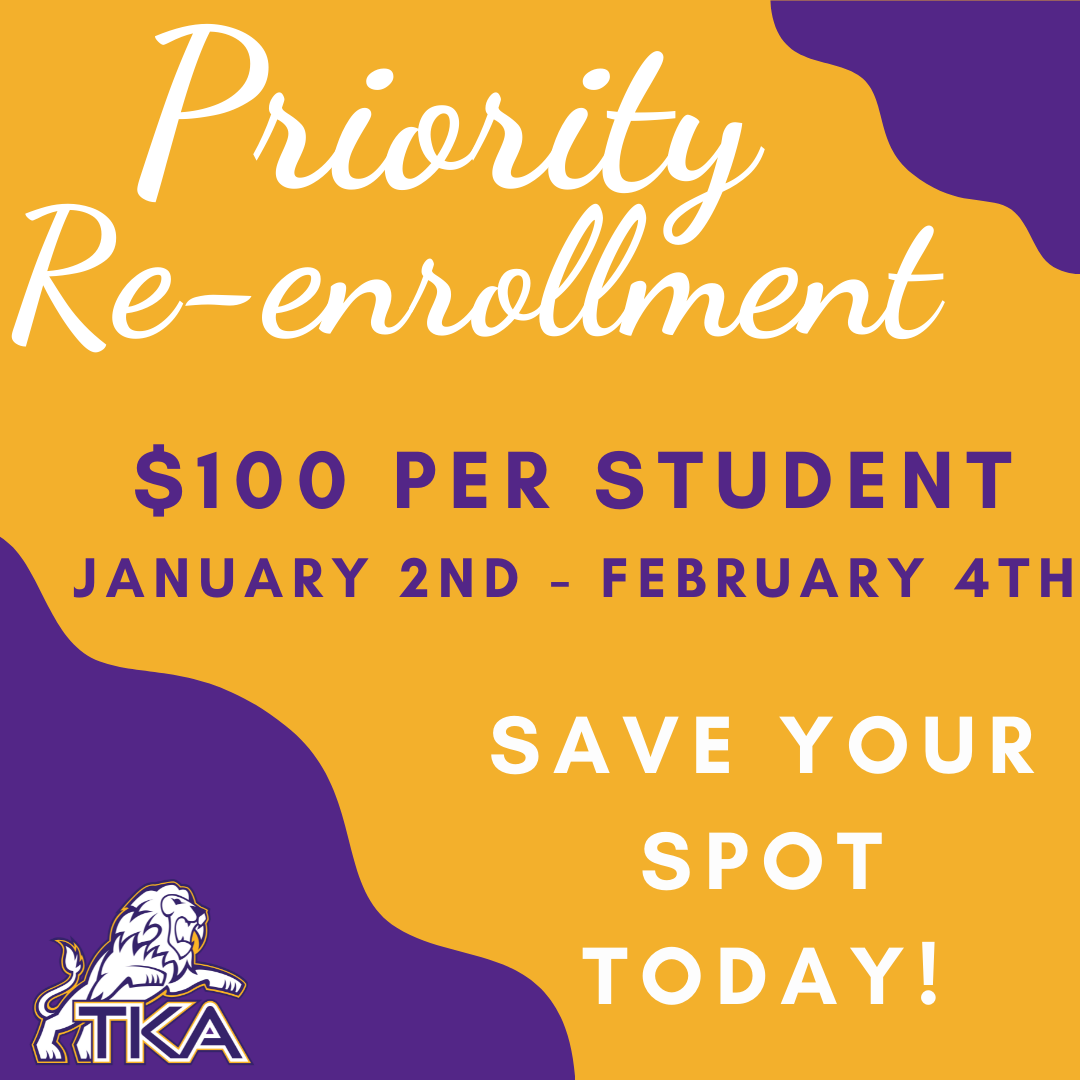# Re-enrollment for 2022-2023

Re-enrollment packets are live in the FACTS Family Portal!

Priority re-enrollment fee of \$100 per student (non-refundable, guarantees your child’s spot) will be from January 2nd – February 4th.

Re-enrollment packets submitted after February 4th will carry a fee of \$300 per student.

The following are to be submitted:
• Re-enrollment form (through FACTS)
• Payment (any of the following options are acceptable):
1. Cash (submitted to either grade level office)
2. Check (submitted to either grade level office)
3. Payment through FACTS account (families will receive more information regarding how to pay this way. This allows for the use of a card or bank draft as a payment option).

If you have a sibling that will be new to TKA for the 2022-2023 school year, please contact Lori Campbell to begin the application process.

Lori Campbell
(865) 573-8321 option 1

 table div table+table+table+table+table+table+table+table div table{width:100%;padding:0}table div table+table+table+table+table+table+table+table div table img{width:96.23%;padding:0;float:none}table div table+table+table+table+table+table+table+table div table td{width:100%;padding:0 1.88% 18px}/* styles */Winter Preview Night for prospective families will be held on Friday, January 21, 2022, at 6:00 in the Brandes Cafeteria. Please refer friends and family who may be interested in learning more about TKA to join us for a night of information, fellowship, and basketball! RSVP by January 19 by clicking here!

 table div table+table+table+table+table+table+table+table+table+table div table{width:100%;padding:0}table div table+table+table+table+table+table+table+table+table+table div table img{width:96.23%;padding:0;float:none}table div table+table+table+table+table+table+table+table+table+table div table td{width:100%;padding:0 1.88% 18px}/* styles */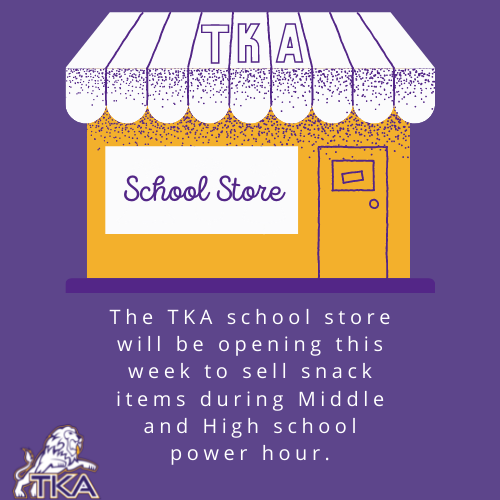# School Store

The TKA school store will be opening this week to sell snack items during Middle and High school power hour.

All snacks that are sold will be under \$5.

 table div table+table+table+table+table+table+table+table+table+table+table+table div table{width:100%;padding:0}table div table+table+table+table+table+table+table+table+table+table+table+table div table img{width:96.23%;padding:0;float:none}table div table+table+table+table+table+table+table+table+table+table+table+table div table td{width:100%;padding:0 1.88% 18px}/* styles */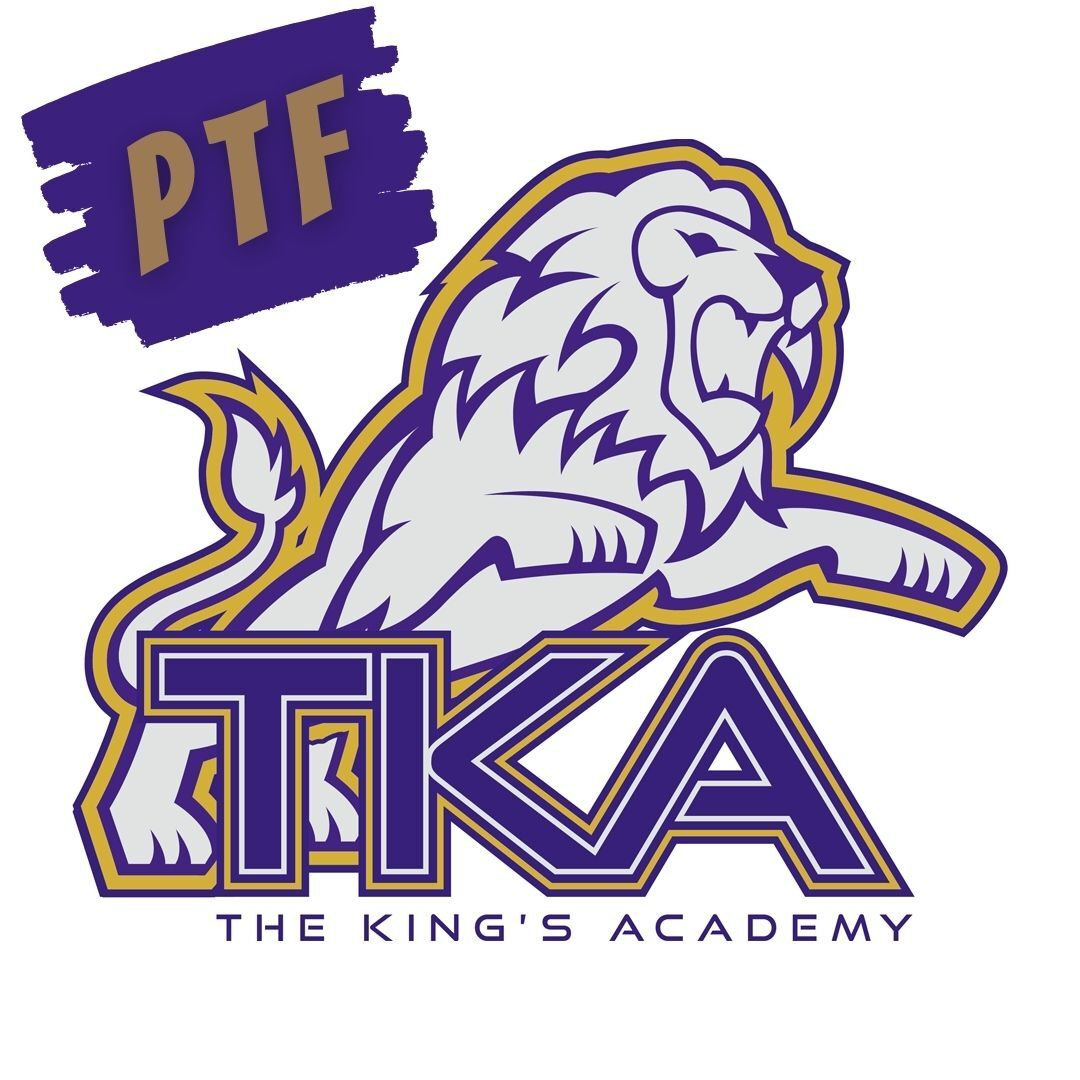# PTF

The next PTF meeting will be tomorrow, January 13, from 3:15 PM-4:05 PM in Brandes.
Mr. Sandefur will be kicking off the New Year in this PTF meeting!

Childcare will be provided throughout the aftercare program free of charge until 4:15 PM for the meeting.

If you haven't joined PTF, no worries, come anyway! Membership forms will be available at the meeting. The fee to join is \$25 per family.

We look forward to having you as part of our PTF this year!

 table div table+table+table+table+table+table+table+table+table+table+table+table+table+table div table{width:100%;padding:0}table div table+table+table+table+table+table+table+table+table+table+table+table+table+table div table img{width:96.23%;padding:0;float:none}table div table+table+table+table+table+table+table+table+table+table+table+table+table+table div table td{width:100%;padding:0 1.88% 18px}/* styles */# Tennis Spring Interest Meeting

High school students who are interested in playing tennis for the spring semester, there will be a meeting on Wednesday, January 19, at 3:15 p.m. in Brandes. No prior experience is needed!

If you are unable to attend or have any questions, email Jenny Cook at lionstkatennis@gmail.com.

 table div table+table+table+table+table+table+table+table+table+table+table+table+table+table+table+table div table{width:100%;padding:0}table div table+table+table+table+table+table+table+table+table+table+table+table+table+table+table+table div table img{width:96.23%;padding:0;float:none}table div table+table+table+table+table+table+table+table+table+table+table+table+table+table+table+table div table td{width:100%;padding:0 1.88% 18px}/* styles */# Upcoming Spring Dress Up Days

Mark your calendars! Jr. Beta will be hosting dress up days each month to continue to partner with and raise funds for the Isaiah 117 House as we continue to Love Where We Live.
For the donation of \$1.00 (or more) per dress up day, students can wear this fun attire. Please remember that all attire must adhere to dress code.

 table div table+table+table+table+table+table+table+table+table+table+table+table+table+table+table+table+table+table div table{width:100%;padding:0}table div table+table+table+table+table+table+table+table+table+table+table+table+table+table+table+table+table+table div table img{width:96.23%;padding:0;float:none}table div table+table+table+table+table+table+table+table+table+table+table+table+table+table+table+table+table+table div table td{width:100%;padding:0 1.88% 18px}/* styles */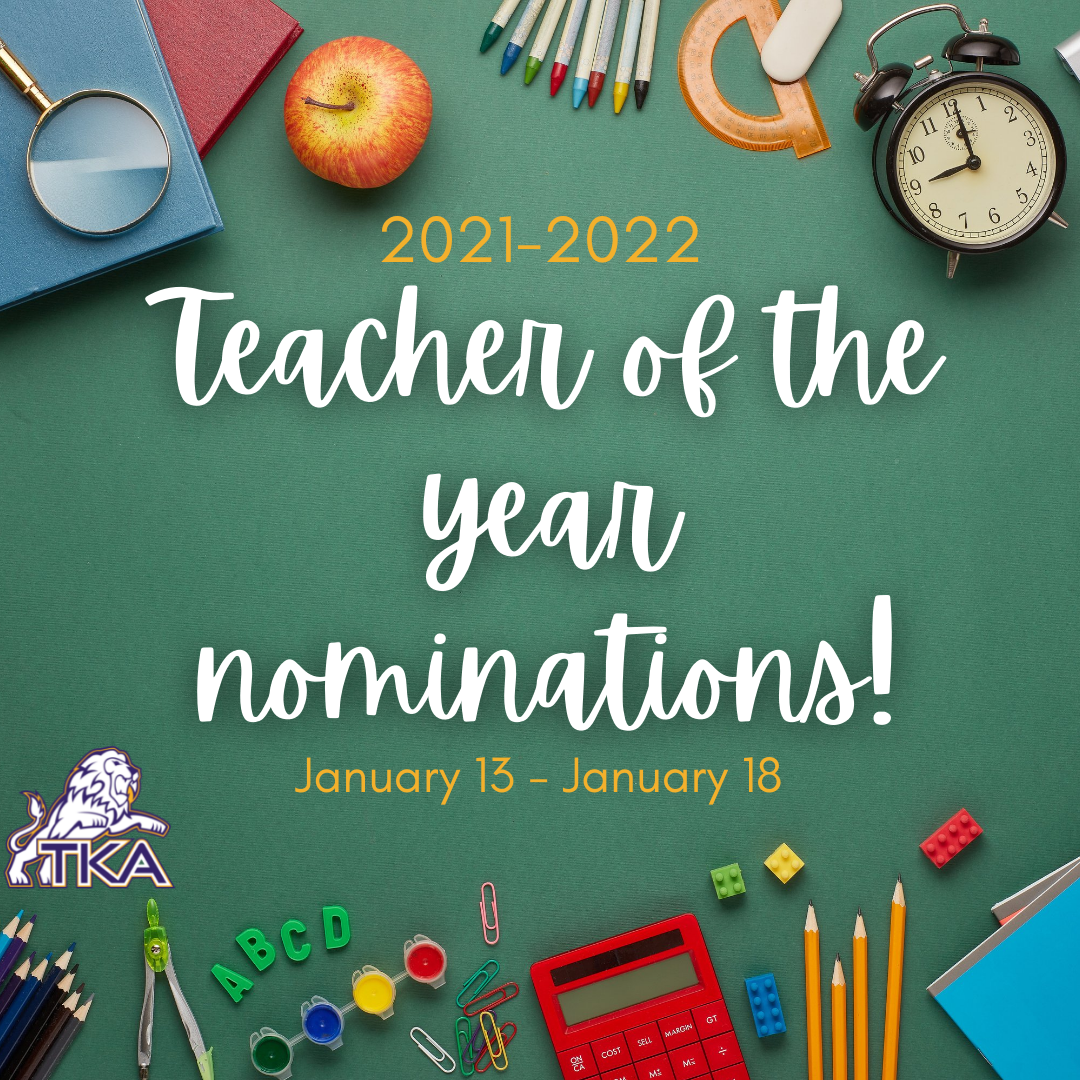# Teacher of the Year Nominations

TKA's 2021-2022 Teacher of the Year will be voted on by students, parents, grandparents, teachers and staff! This award will be given to one elementary teacher and one middle/high school teacher who has made an impact reaching beyond his/her classroom at TKA.

Nominations will begin on Thursday, January, 13, and end on Tuesday, January 18, at 3:00pm. Nomination forms can be downloaded with this link and can be picked up in both front offices. One nomination per person please.

All nomination forms should be filled out and turned into a ballot box located in either the elementary school front office or the middle/high school front office by January 18th at 3:00 p.m.

 table div table+table+table+table+table+table+table+table+table+table+table+table+table+table+table+table+table+table+table+table div table{width:100%;padding:0}table div table+table+table+table+table+table+table+table+table+table+table+table+table+table+table+table+table+table+table+table div table img{width:96.23%;padding:0;float:none}table div table+table+table+table+table+table+table+table+table+table+table+table+table+table+table+table+table+table+table+table div table td{width:100%;padding:0 1.88% 18px}/* styles */# Love Gala

You're invited to the first annual Love Gala hosted by The King's Academy. The Love Gala will be on February 12, 2022, at Johnson University in Knoxville, TN. Plan on joining us for a night of fellowship, an exciting auction with great items, and a time to honor our alumni, teachers, and those who have left a legacy at TKA.

Each ticket purchased for the event will have an opportunity to win FREE tuition for one student for the 2022-23 academic year. Additional tuition remission opportunity tickets are available for purchase at the door for \$25 per opportunity. All opportunity drawing tickets are Non-Transferable. Only the student drawn is eligible for tuition remission. Must be present to receive tuition remission.To purchase tickets and RSVP for the event, scan this QR Code.

 table div table+table+table+table+table+table+table+table+table+table+table+table+table+table+table+table+table+table+table+table+table+table+table div table{width:100%;padding:0}table div table+table+table+table+table+table+table+table+table+table+table+table+table+table+table+table+table+table+table+table+table+table+table div table img{width:96.23%;padding:0;float:none}table div table+table+table+table+table+table+table+table+table+table+table+table+table+table+table+table+table+table+table+table+table+table+table div table td{width:100%;padding:0 1.88% 18px}/* styles */# Elementary Spring Clubs

TKA Elementary Families,

We are excited to announce that our TKA Elementary spring club opportunities will begin very soon. The spring section will begin the week of January 31st and end April 22nd.

Each club will include a \$20 participation fee to cover resources needed for club activities. Unfortunately, each club has a maximum number of 20 students. Our STEAM and Whiz Kidz Clubs will have a maximum number of 15 students.

Please click on a club to review the club description and registration link. Again, we will select the first participants until the maximum number is reached. For spring clubs, new participants in club areas will be selected first prior to returning students who participated in the fall semester. We ask that you consider all options and register for clubs that fit your schedule for the year. To be fair to our club instructors, we ask that you choose a club where your child will be able to participate and attend 90% of the semester club activities.

All sign up forms will close Friday, January 21st. Our club teachers will be in communication with participant families for their club area by Friday, January 28th to discuss first meeting details and next steps.

We are very blessed to have such wonderful teachers who are excited to provide these enriching opportunities after school hours to further enhance our elementary learning.

Blessings,
Kelly SullivanBook Club will provide students with the opportunity to engage with texts deeply in a relaxed fun environment. Through group discussion and hands-on activities, students will practice age appropriate comprehension skills such as character analysis, distinguishing literary themes, understanding the elements of plot development, and using textual evidence. Book club will also provide time for readers to share book recommendations with each other, through book talks and book lists, in order to deepen their self-knowledge as a reader and broaden their range of choice in reading material. Reading choice is vital to developing a lifelong love of reading. Choice of club texts will be determined in the first few meetings of the club so readers can have input and ownership of their experience.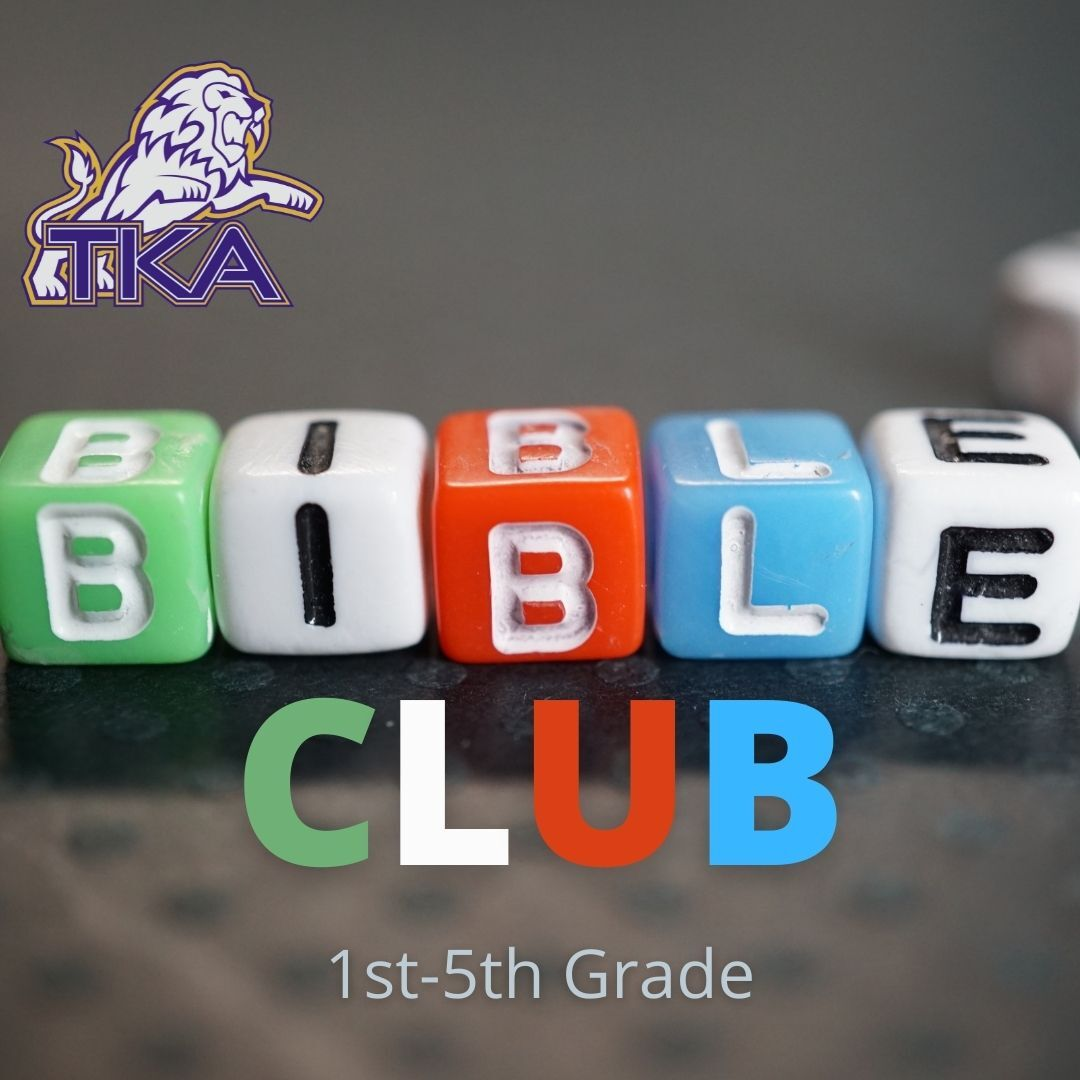Bible Club will include Bible Drill, Memory Verses, Bible Story Interacting (drawing, drama, games, questions, songs,) . . . and Developing a Personal Quiet Time.# K4-K5 (Grades K4-K5) STEAM Club

K4-K5 STEAM Club will include a variety of hands-on activities focusing on science, technology, engineering, arts, and mathematics that are age appropriate for our little lions.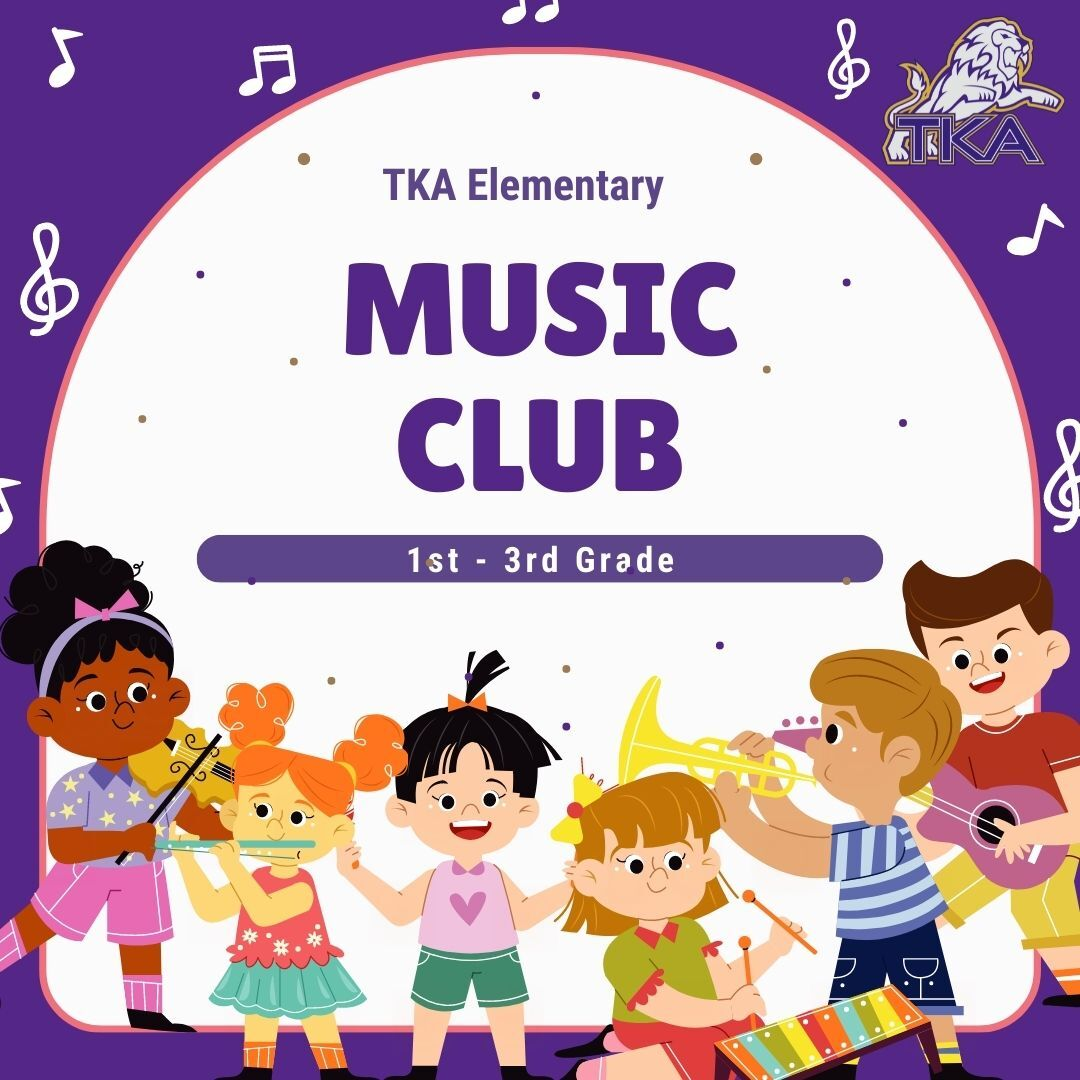# Music Club (Grades 1-3 only)

Join us as we Create, Perform, and Respond to music in lots of fun ways! A few of the activities we will be doing:

▪ Singing
▪ Playing instruments
▪ Moving to music
▪ Listening to music
▪ Composing, arranging, and performing music
 ▪ Singing
 ▪ Playing instruments
 ▪ Moving to music
 ▪ Listening to music
 ▪ Composing, arranging, and performing music# Whiz Kidz (3-5 grades only)

This STEAM Club will include a variety of hands-on activities focusing on science, technology, engineering, arts, and mathematics that are age appropriate for our intermediate lions.

 table div table+table+table+table+table+table+table+table+table+table+table+table+table+table+table+table+table+table+table+table+table+table+table+table+table+table+table+table+table+table div table{width:100%;padding:0}table div table+table+table+table+table+table+table+table+table+table+table+table+table+table+table+table+table+table+table+table+table+table+table+table+table+table+table+table+table+table div table img{width:96.23%;padding:0;float:none}table div table+table+table+table+table+table+table+table+table+table+table+table+table+table+table+table+table+table+table+table+table+table+table+table+table+table+table+table+table+table div table td{width:100%;padding:0 1.88% 18px}/* styles */# Elementary STEAM Week 2022

This year we will host our 2nd Annual STEAM (Science, Technology, Engineering, Arts, Math) Week for our students during regular school hours the week of January 24-28.

During this special week, a focus on STEAM will permeate the entire elementary school! Elementary classroom doors and hallways will feature STEAM-themed decorations, daily science classes will include special STEAM activities, and even enhancement classes (PE, Music, Art, and Library) will integrate our STEAM focus into their instruction! Also, students will have access to our very own STEAM Store (select hours available) to purchase fun science items (pencils, notepads, folders, erasers, and more) along with a variety of snack items for the cost of \$1 each.

Ms. Sullivan will be sending more information to Elementary parents regarding this special week. We are looking forward to an exciting and engaging STEAM Week!

 table div table+table+table+table+table+table+table+table+table+table+table+table+table+table+table+table+table+table+table+table+table+table+table+table+table+table+table+table+table+table+table+table div table{width:100%;padding:0}table div table+table+table+table+table+table+table+table+table+table+table+table+table+table+table+table+table+table+table+table+table+table+table+table+table+table+table+table+table+table+table+table div table img{width:96.23%;padding:0;float:none}table div table+table+table+table+table+table+table+table+table+table+table+table+table+table+table+table+table+table+table+table+table+table+table+table+table+table+table+table+table+table+table+table div table td{width:100%;padding:0 1.88% 18px}/* styles */WHO: PreK3-5th grade students and their Dad (Grandfather, Uncle, or other important male figure)

WHEN: Saturday, February 19, from 6p.m. to 8 p.m.

WHERE: Sevierville Civic Center (200 Gary R. Wade Blvd)

COST: \$90 per couple/\$45 per additional child (sorry, no refunds) The price includes Dinner and Photo.

For any questions regarding this event, please email Summer Delius at summerdelius@gmail.com.

 table div table+table+table+table+table+table+table+table+table+table+table+table+table+table+table+table+table+table+table+table+table+table+table+table+table+table+table+table+table+table+table+table+table+table div table{width:100%;padding:0}table div table+table+table+table+table+table+table+table+table+table+table+table+table+table+table+table+table+table+table+table+table+table+table+table+table+table+table+table+table+table+table+table+table+table div table img{width:96.23%;padding:0;float:none}table div table+table+table+table+table+table+table+table+table+table+table+table+table+table+table+table+table+table+table+table+table+table+table+table+table+table+table+table+table+table+table+table+table+table div table td{width:100%;padding:0 1.88% 18px}/* styles */# Upcoming Weekly Sporting Events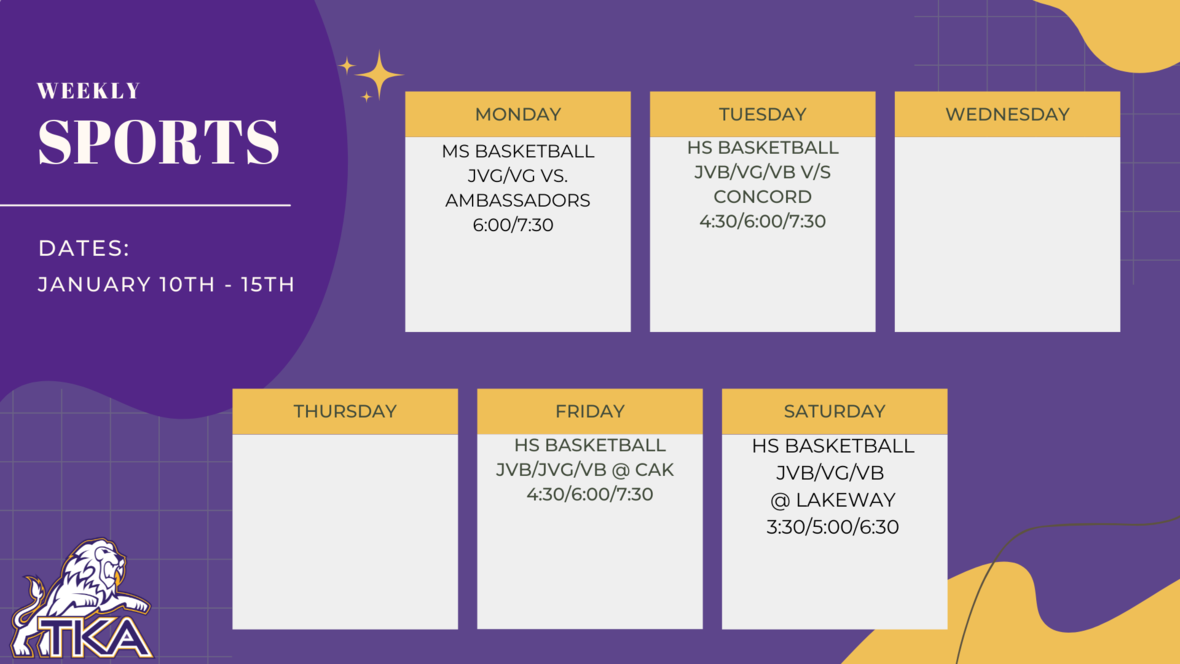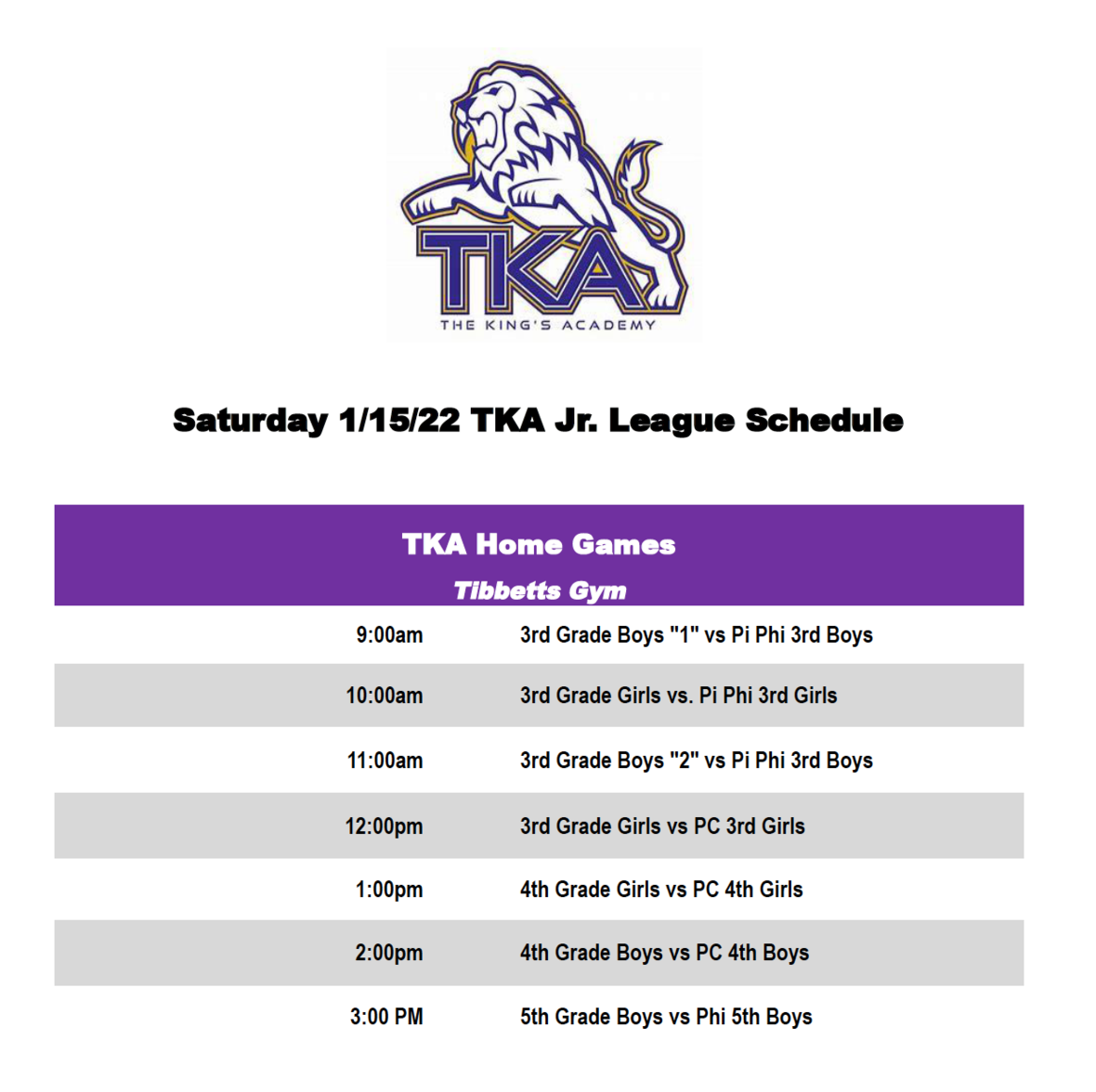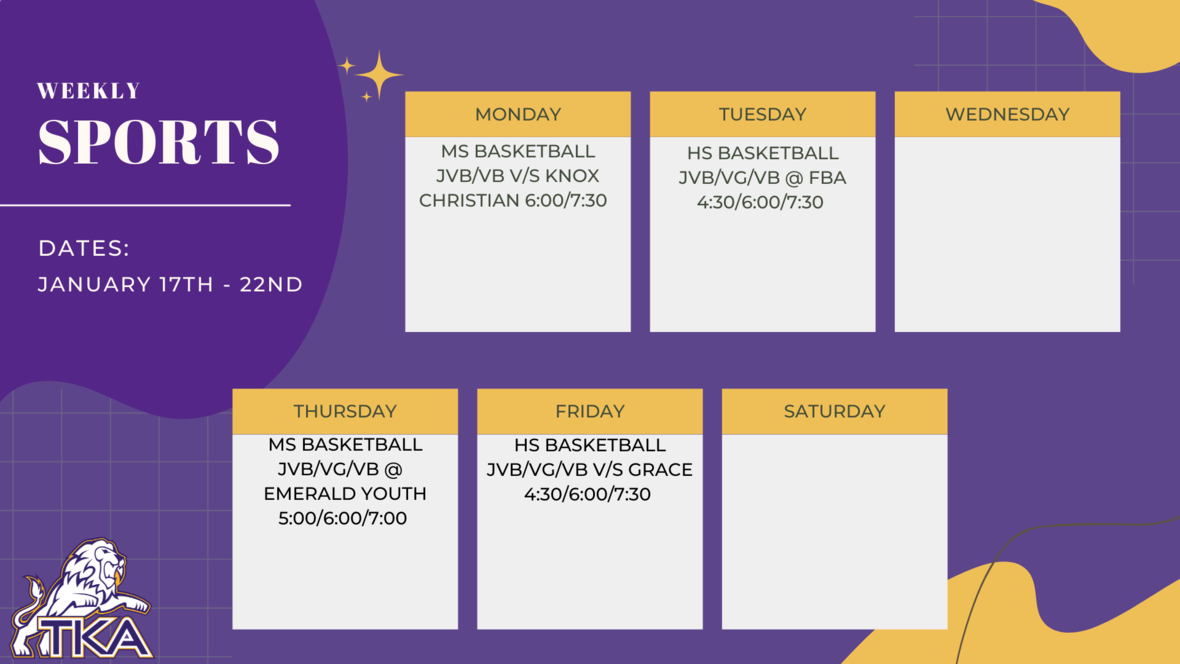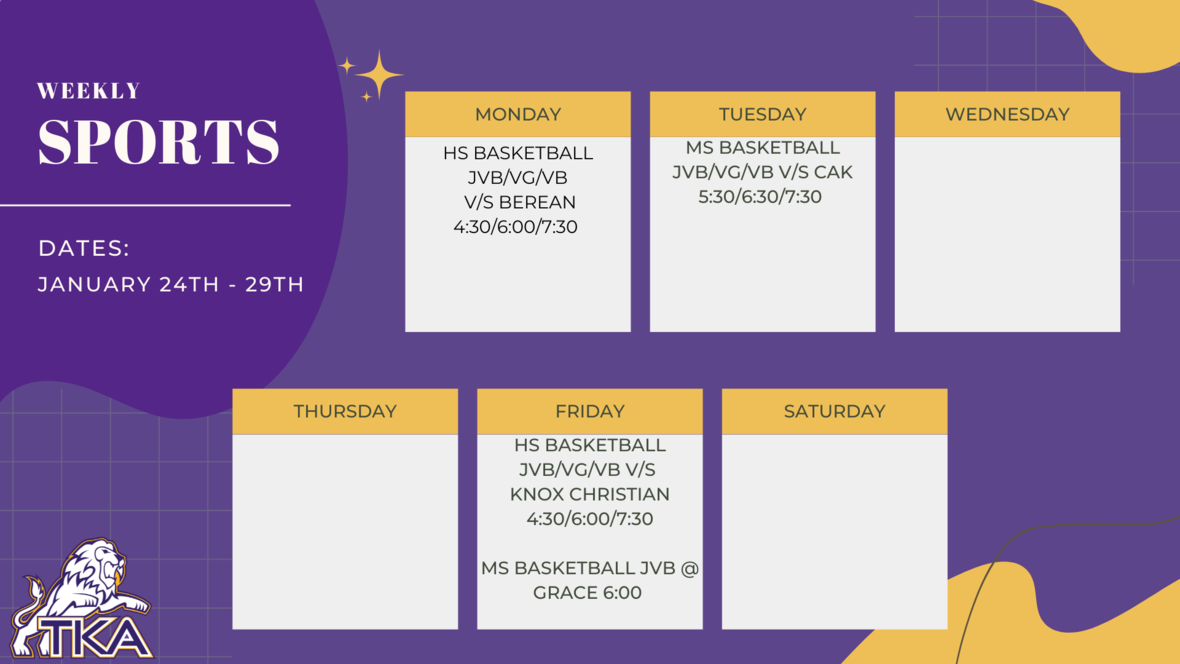table div table+table+table+table+table+table+table+table+table+table+table+table+table+table+table+table+table+table+table+table+table+table+table+table+table+table+table+table+table+table+table+table+table+table+table+table+table+table+table+table div table{width:100%;padding:0}table div table+table+table+table+table+table+table+table+table+table+table+table+table+table+table+table+table+table+table+table+table+table+table+table+table+table+table+table+table+table+table+table+table+table+table+table+table+table+table+table div table img{width:96.23%;padding:0;float:none}table div table+table+table+table+table+table+table+table+table+table+table+table+table+table+table+table+table+table+table+table+table+table+table+table+table+table+table+table+table+table+table+table+table+table+table+table+table+table+table+table div table td{width:100%;padding:0 1.88% 18px}/* styles */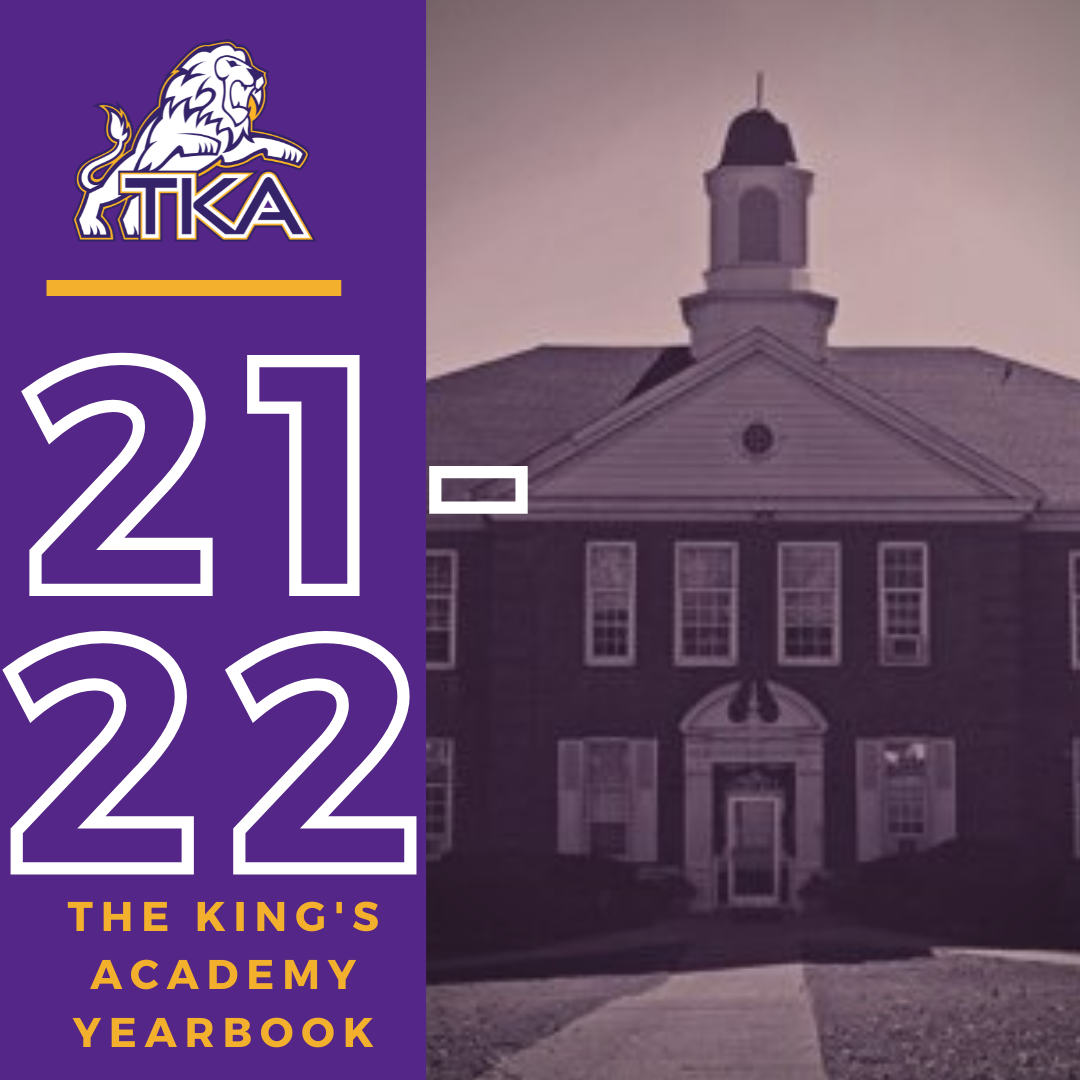# 2021-2022 Yearbook

The price for the 2021-2022 TKA yearbook is \$55. All grades, K3-12 will have the same yearbook. The slight price increase is because we are only going to have one larger all-school book.

All yearbook orders can be placed online--here is the link.

Senior Tributes and Business ads are available for purchase at the bottom of the link listed here.

For any questions regarding yearbooks, email Mr. Josh Smith at jsmith@thekingsacademy.net.

 table div table+table+table+table+table+table+table+table+table+table+table+table+table+table+table+table+table+table+table+table+table+table+table+table+table+table+table+table+table+table+table+table+table+table+table+table+table+table+table+table+table+table div table{width:100%;padding:0}table div table+table+table+table+table+table+table+table+table+table+table+table+table+table+table+table+table+table+table+table+table+table+table+table+table+table+table+table+table+table+table+table+table+table+table+table+table+table+table+table+table+table div table img{width:96.23%;padding:0;float:none}table div table+table+table+table+table+table+table+table+table+table+table+table+table+table+table+table+table+table+table+table+table+table+table+table+table+table+table+table+table+table+table+table+table+table+table+table+table+table+table+table+table+table div table td{width:100%;padding:0 1.88% 18px}/* styles *//* styles */
 table div table+table+table+table+table+table+table+table+table+table+table+table+table+table+table+table+table+table+table+table+table+table+table+table+table+table+table+table+table+table+table+table+table+table+table+table+table+table+table+table+table+table+table+table+table div table{width:100%;padding:0}table div table+table+table+table+table+table+table+table+table+table+table+table+table+table+table+table+table+table+table+table+table+table+table+table+table+table+table+table+table+table+table+table+table+table+table+table+table+table+table+table+table+table+table+table+table div table img{width:96.23%;padding:0;float:none}table div table+table+table+table+table+table+table+table+table+table+table+table+table+table+table+table+table+table+table+table+table+table+table+table+table+table+table+table+table+table+table+table+table+table+table+table+table+table+table+table+table+table+table+table+table div table td{width:100%;padding:0 1.88% 18px}/* styles */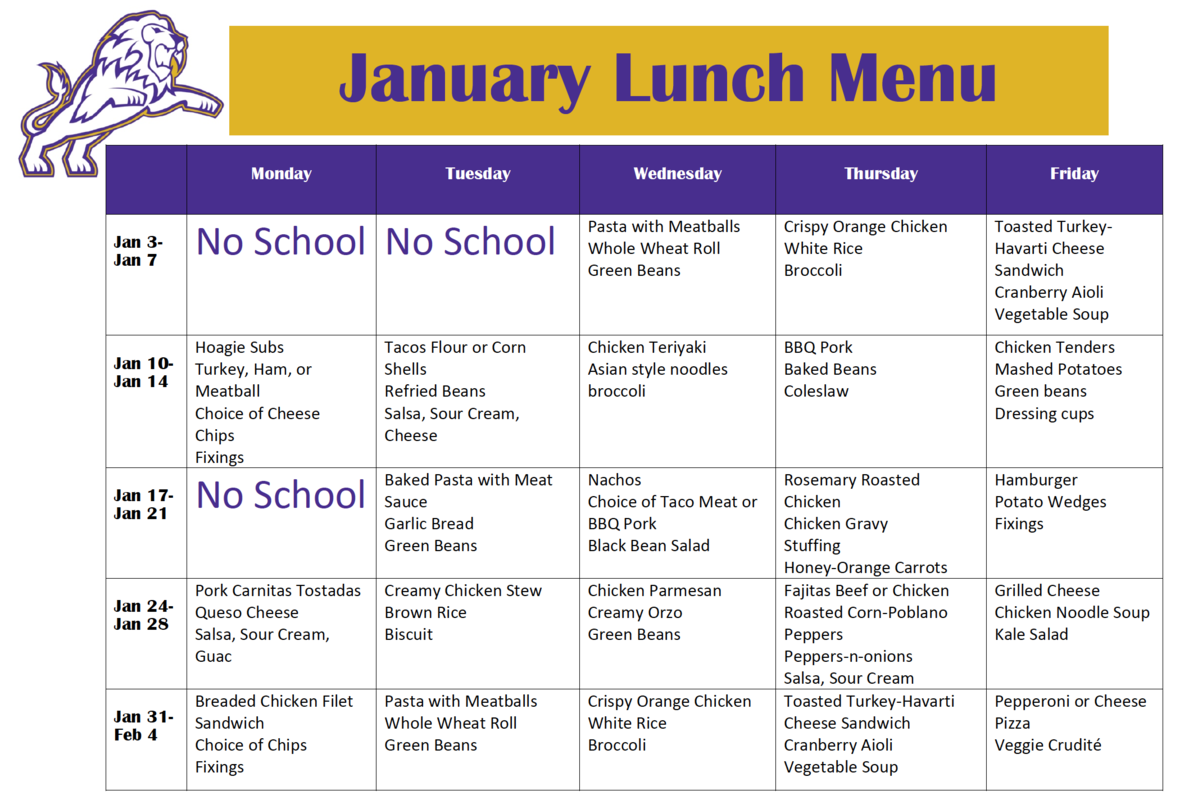table div table+table+table+table+table+table+table+table+table+table+table+table+table+table+table+table+table+table+table+table+table+table+table+table+table+table+table+table+table+table+table+table+table+table+table+table+table+table+table+table+table+table+table+table+table+table+table div table{width:100%;padding:0}table div table+table+table+table+table+table+table+table+table+table+table+table+table+table+table+table+table+table+table+table+table+table+table+table+table+table+table+table+table+table+table+table+table+table+table+table+table+table+table+table+table+table+table+table+table+table+table div table img{width:96.23%;padding:0;float:none}table div table+table+table+table+table+table+table+table+table+table+table+table+table+table+table+table+table+table+table+table+table+table+table+table+table+table+table+table+table+table+table+table+table+table+table+table+table+table+table+table+table+table+table+table+table+table+table div table td{width:100%;padding:0 1.88% 18px}/* styles */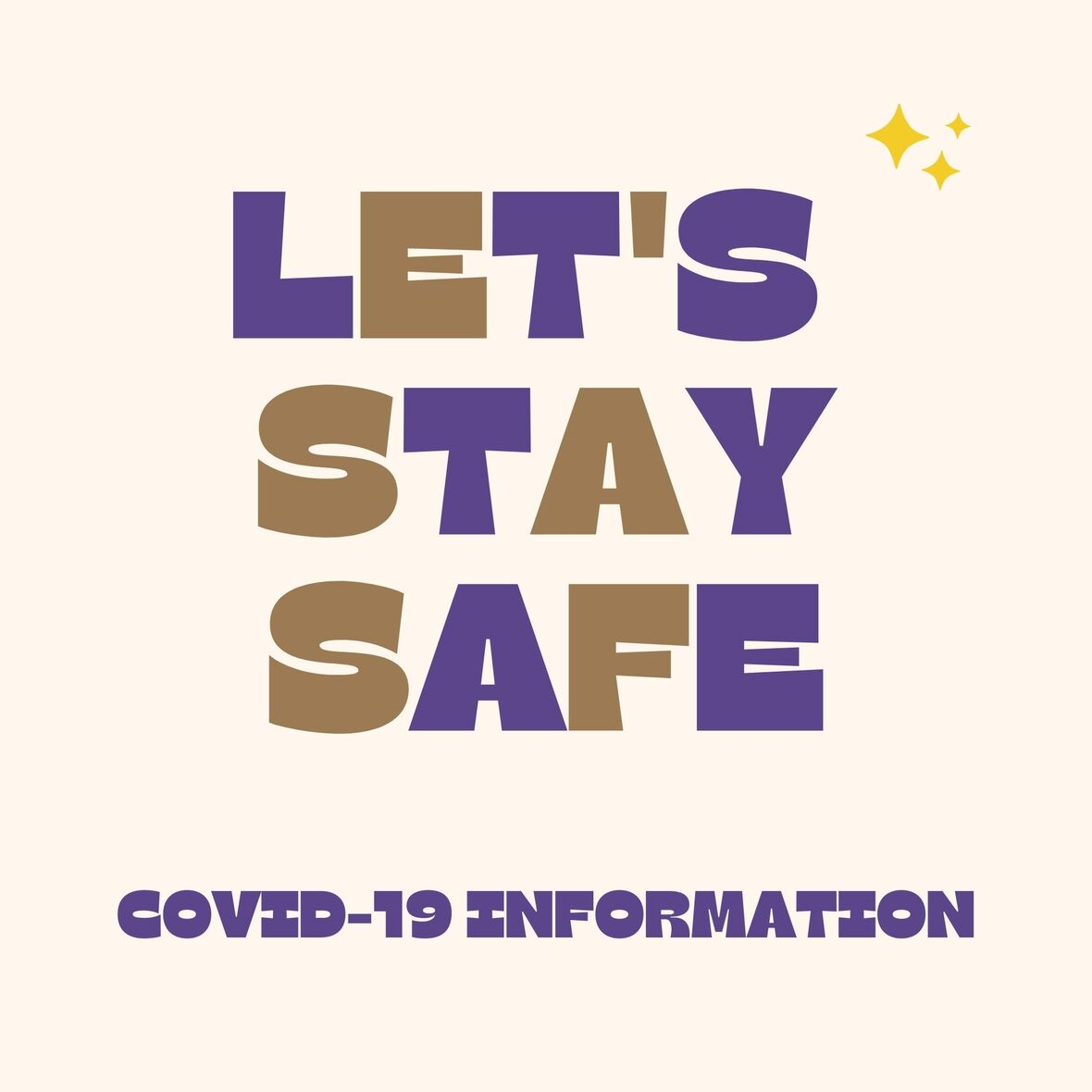# Covid-19 Procedures

While we are excited to offer in-person education, we must maintain a safe learning environment for all students, faculty/staff, and additional stakeholders.

To do so, we will be working closely with the Sevier County Health Department regarding Covid-19 protocols.

 table div table+table+table+table+table+table+table+table+table+table+table+table+table+table+table+table+table+table+table+table+table+table+table+table+table+table+table+table+table+table+table+table+table+table+table+table+table+table+table+table+table+table+table+table+table+table+table+table+table div table{width:100%;padding:0}table div table+table+table+table+table+table+table+table+table+table+table+table+table+table+table+table+table+table+table+table+table+table+table+table+table+table+table+table+table+table+table+table+table+table+table+table+table+table+table+table+table+table+table+table+table+table+table+table+table div table img{width:96.23%;padding:0;float:none}table div table+table+table+table+table+table+table+table+table+table+table+table+table+table+table+table+table+table+table+table+table+table+table+table+table+table+table+table+table+table+table+table+table+table+table+table+table+table+table+table+table+table+table+table+table+table+table+table+table div table td{width:100%;padding:0 1.88% 18px}/* styles */table div table+table+table+table+table+table+table+table+table+table+table+table+table+table+table+table+table+table+table+table+table+table+table+table+table+table+table+table+table+table+table+table+table+table+table+table+table+table+table+table+table+table+table+table+table+table+table+table+table+table+table div table{width:100%;padding:0}table div table+table+table+table+table+table+table+table+table+table+table+table+table+table+table+table+table+table+table+table+table+table+table+table+table+table+table+table+table+table+table+table+table+table+table+table+table+table+table+table+table+table+table+table+table+table+table+table+table+table+table div table img{width:96.23%;padding:0;float:none}table div table+table+table+table+table+table+table+table+table+table+table+table+table+table+table+table+table+table+table+table+table+table+table+table+table+table+table+table+table+table+table+table+table+table+table+table+table+table+table+table+table+table+table+table+table+table+table+table+table+table+table div table td{width:100%;padding:0 1.88% 18px}/* styles */